12 Dec

The mathematical purpose of this project is to explore the geometry of the piles that form when sand is poured on different 2D shapes. Specifically, we investigate the ridges and apexes that form on these piles. In the case of the polygonal shapes, we define a ridge as where two planes meet at a line, and an apex as where three or more planes meet at a point. We decided to focus on these aspects, and particularly their projections onto the 2D shape beneath them because they are easier to analyze in 2D, and because the actual angle the pile of sand slopes at depends on the sand itself, and not on the geometry of the shape it is on.

First, we define what convex/concave means. Consider any vertex of a polygon. Then look at the two vertices at the ends of the two edges that form the original vertex. If the line drawn between these two vertices lies at least partially inside of the polygon, then define the original vertex as a convex vertex. If the line lies entirely outside of the polygon, then define the original vertex as a concave vertex.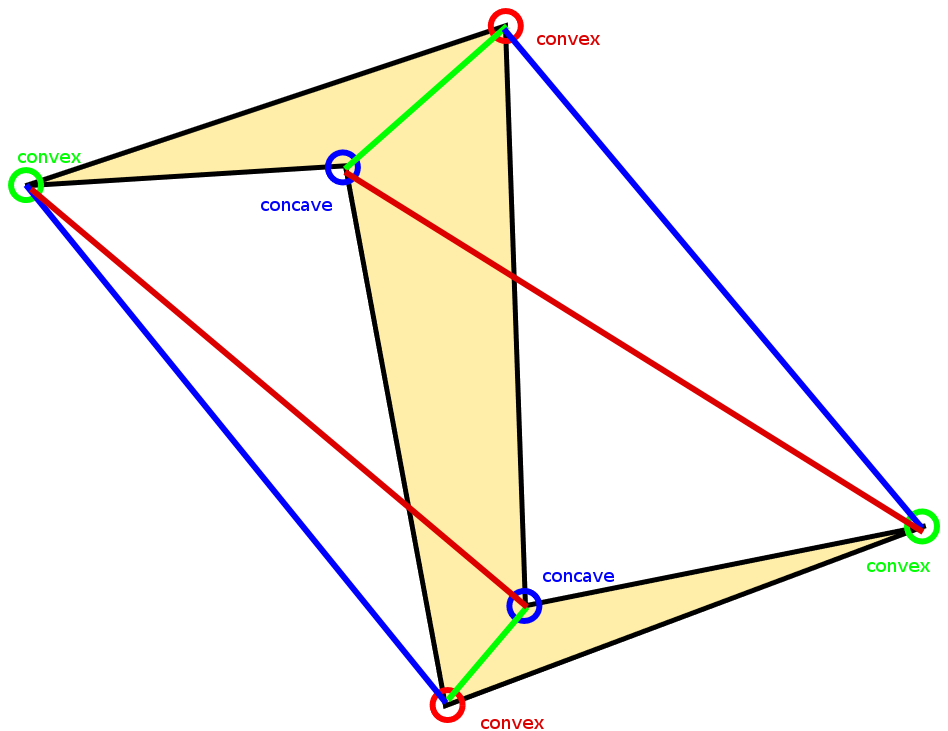The main observation that we came to and hope that participants will gain intuition for is that, given a polygon, the ridges when projected onto the shape are the angle-bisectors of each convex vertex, and the apexes occur where these bisectors meet. Once they meet at an apex, the bisectors disappear and are replaced with a single new ridge that connects to another apex (this is particularly visible in the case of the rectangle). The logic behind this phenomenon is that a convex vertex produce ridges because sand resting on top of the shape needs to minimize the distance between its two forming edges, whereas with a concave vertex, its two forming edges create negative space where sand cannot rest.

Note that concave vertices do not create ridges or affect other ridges, which is exemplified by the the fact that the triangle and the concave hexagon have the same ridges. The logic behind this phenomenon is that a convex vertex produce ridges because sand resting on top of the shape needs to fall in the middle of two edges, whereas with a concave vertex, its two forming edges create negative space where sand cannot rest.

While the curved shapes appear to be more intimidating, they are in fact governed by the same principle: the “ridge” is the set of points equidistant to the two closet edges. In the cases of polygons, this set is the angle bisector.

In the case of the curved plates, this definition defines conic sections. For the circular plate with the circular hole in the center, the set of points exactly halfway between the two circles in a circle exactly halfway between the other two. For the off-center circle, this same process makes what looks like a circle in 2D, but is actually an ellipse in 3D. Lastly, the small hole on the rectangular plate simulates part of a parabola, since a parabola is defined to be the set of points equidistant to a point and and line (and here we approximate a point as a small circle, so sand can fall through).

30 Sep

The inspiration for this blog post is a plain breakfast bagel, as pictured below in this photo taken in Winthrop Dining Hall on a Sunday morning. The bagel closely resembles a shape very important in the topics of geometry and topology in mathematics–that is, the torus.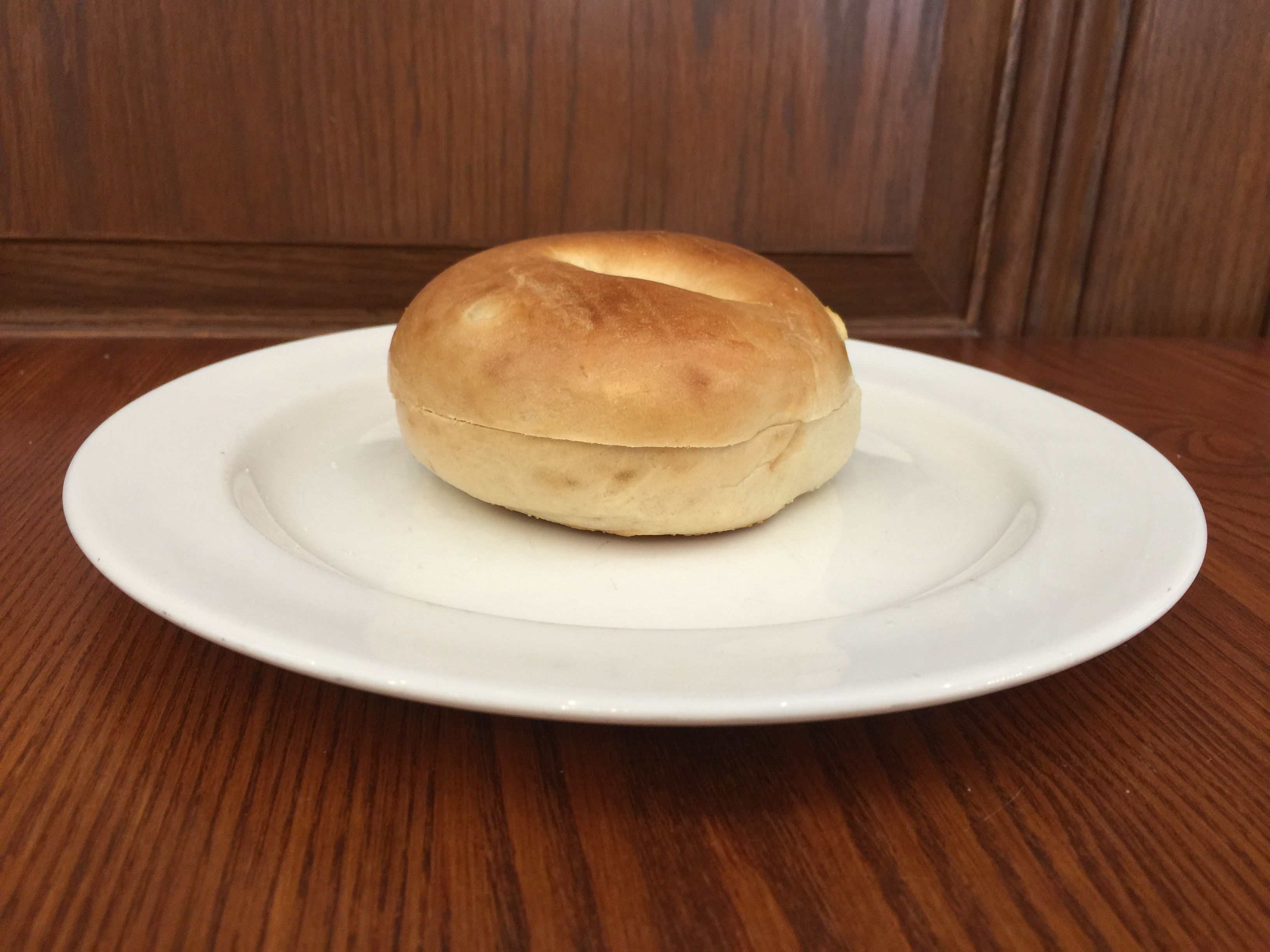The Bagel and the Torus
The torus is a surface which you can picture as follows: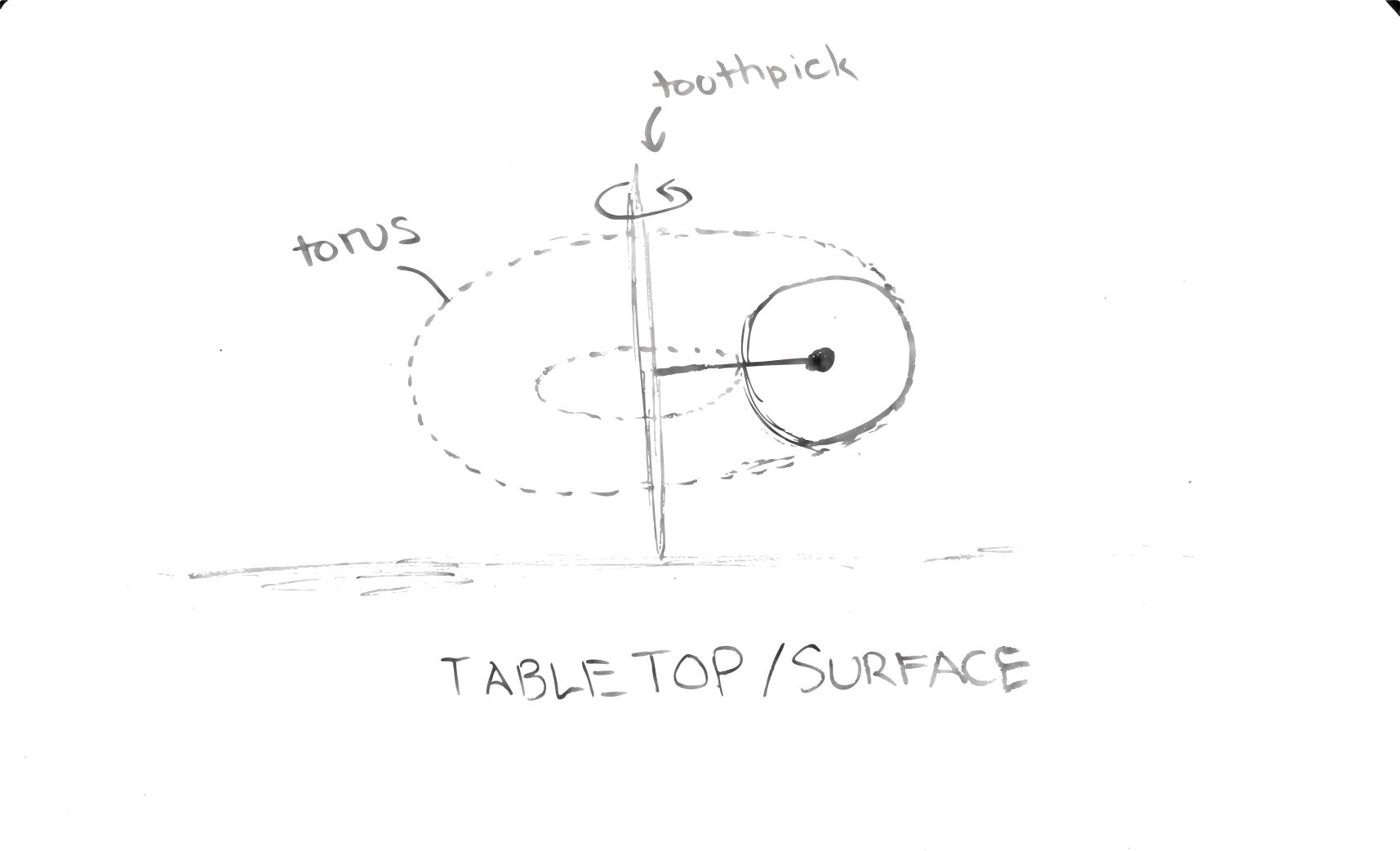Tori can live in any n-dimensional space for n>2, but because humans view bagels in 3-D, this discussion will center around the torus constructed in 3-dimensional space.

One way to visualize the torus is as follows:

• Consider a toothpick held vertically against a tabletop. This will be our axis of rotation.
• For a circle drawn on the same plane as the line passing through the toothpick, the surface created by spinning the toothpick 360 degrees forms the entire torus.

However, bagels are not hollow. Therefore, the rest of this discussion will focus on the solid torus, whose surface is an ordinary torus like the one visualized in the steps above, but also includes all of the points trapped within the torus.

Even acknowledging that real bagels are imperfect and therefore only closely approximates the solid torus, we see that this seemingly mundane and rather plain breakfast item becomes a foundational example in the field of topology!

Cutting The Bagel

If you enjoy your morning bagel with cream cheese, then some interesting mathematics might come into play: How should I slice my bagel so that I can have more space to spread cream cheese?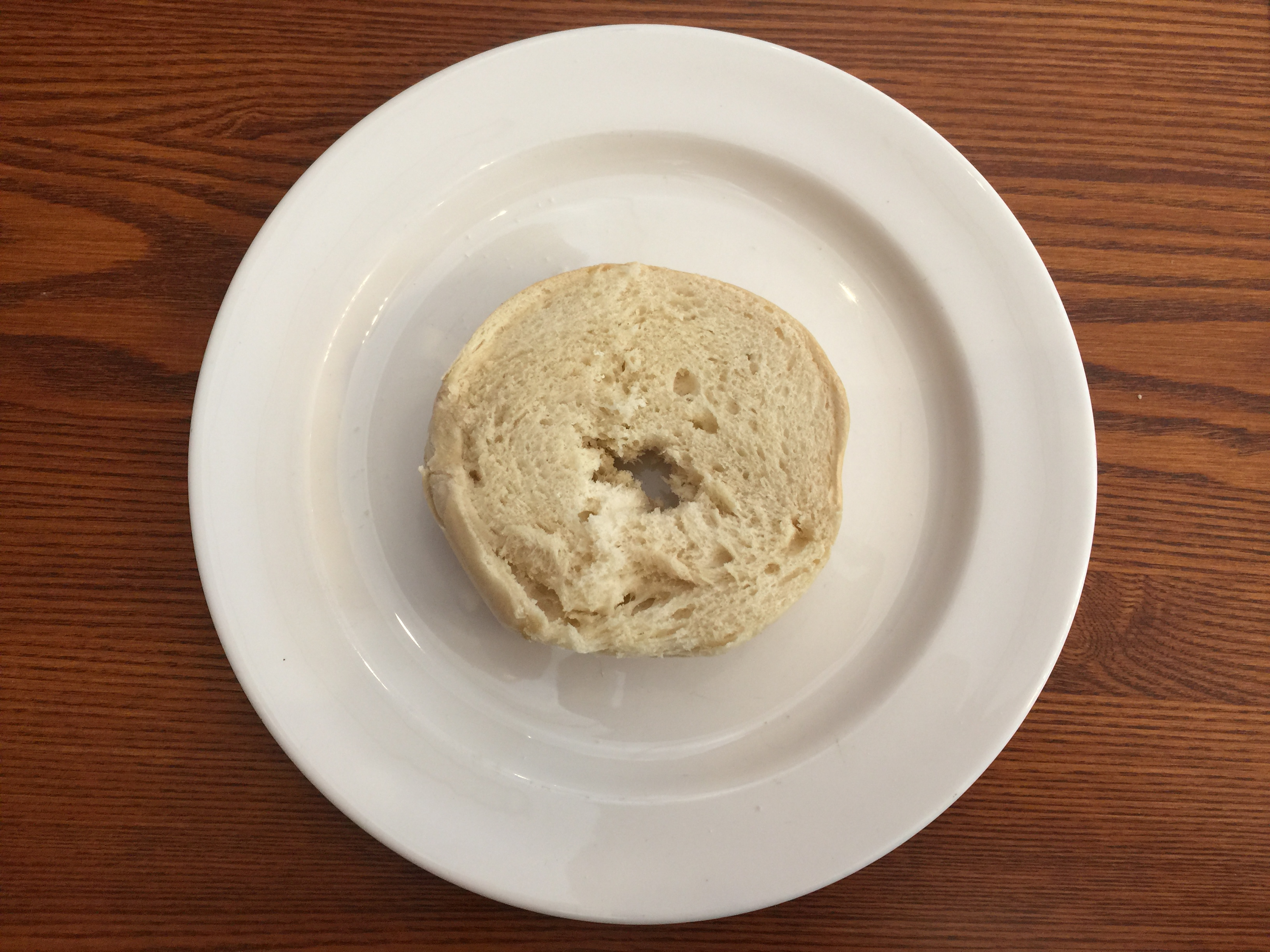Typically, the bagel is sliced horizontally to create two halves on which to spread cream cheese. Let us say we will limit the slicing to one connected motion of a knife.

What if we slice using a cut shaped like a sine wave all around the bagel? Surely that would give more surface area of exposed white bread for cream cheese.Expanding this to an extreme case, what if we keep increasing the frequency of this sine wave so that we have a near-infinite number of ridges on which to spread my cream cheese? Continuing this logic, in theory there is no limit to the amount of surface area we can generate with the cut. Therefore this question becomes difficult to answer. Perhaps the best way to maximize the surface area of exposed bagel is to just cut as many ridges as physically possible with my knife along the side of my bagel, where the limit of how thin we can slice is only physical reality.

An Interesting Cut

In 2012, Professor George W. Hart published an article about an activity he introduced to his students at Stony Brook University in which they were challenged with slicing a bagel into congruent, interlocking halves in one cut.

If you want to give this puzzle a shot, then put a hold on reading this blog post and find yourself a bagel and a knife.

.

.

.

The solution to this puzzling task is to slice the bagel along a two-twist Mobius Strip. (https://www.georgehart.com/bagel/bagel.html)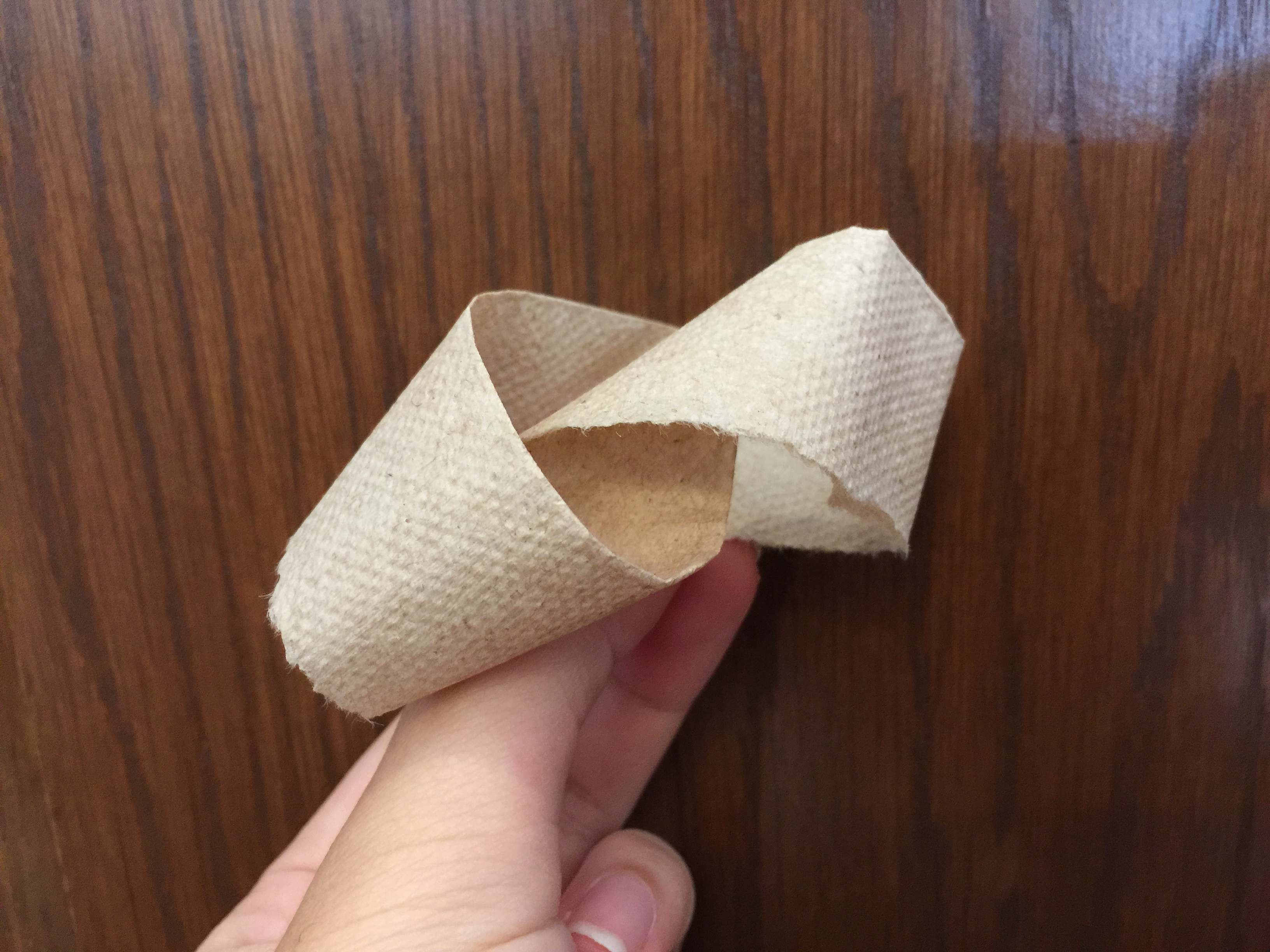*One great way to visualize a two-twist Mobius Strip is to take a strip of paper, turn one end around twice while holding the other still, and gluing the two ends together.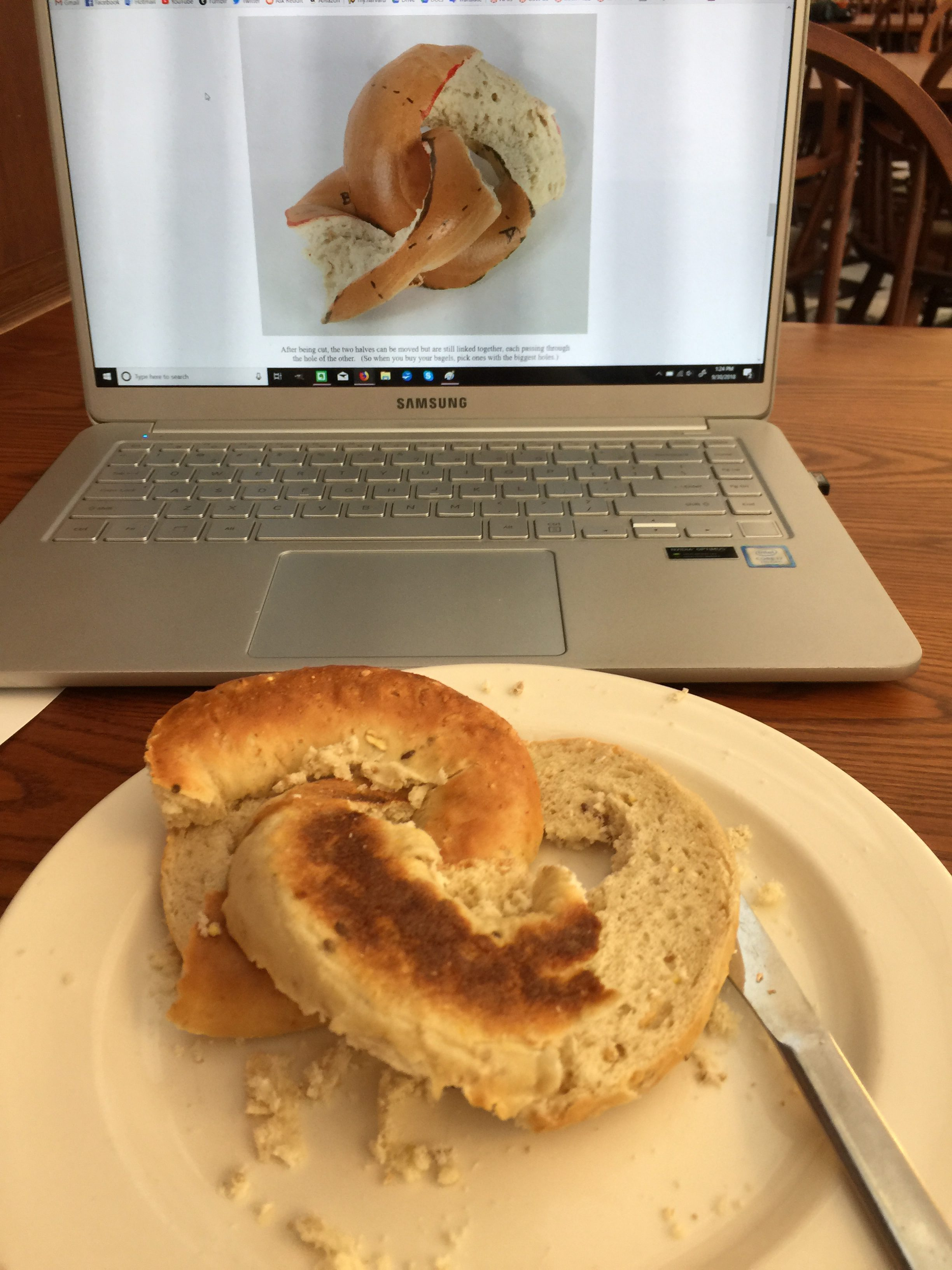I bring this up because Professor Hart’s challenge to his students is a fun and engaging activity that draws the attention of people who may never have even heard of tori and mobius strips. The idea of a single cut that splits one ring into two interlocking ones is exciting, and for that reason I will use this specific cut as an example in the exploration of surface area. Does this cut increase the amount of exposed bagel on which I can spread cream cheese? While this is surely not the most time-efficient method of cutting a bagel, we can use mathematics to test whether or not such a cut would actually give us more space on which we can slather our tasty cream cheese.

Calculating the Surface Area of Exposed Bagel in the Mobius Cut

In order to calculate the surface area of exposed bagel for our cream cheese, we will have to introduce some calculus: First, we will parametrize the torus using the following parametrization (in other words, re-representing the torus using a different set of variables):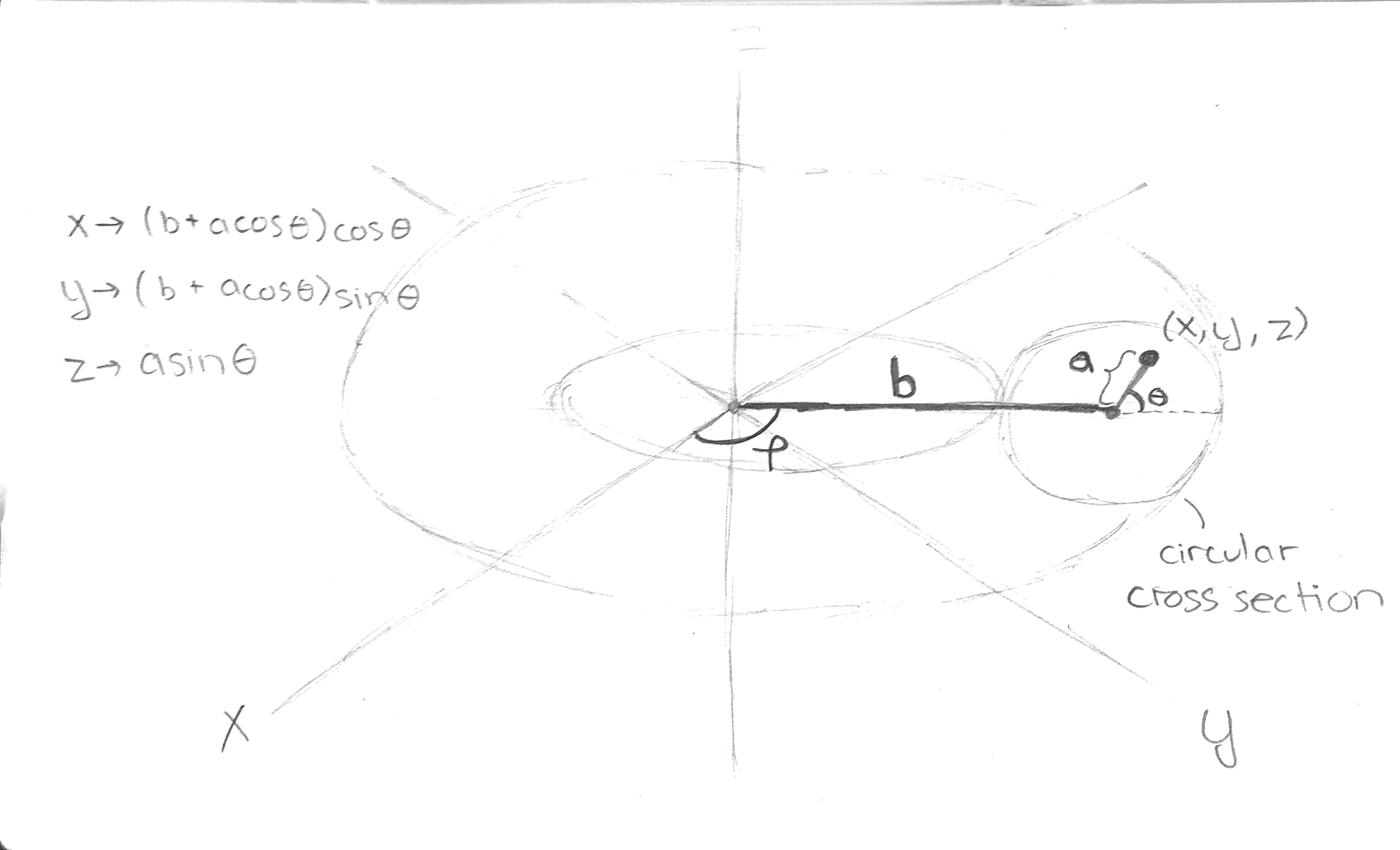The parametrization is (x,y,z) = P(a, θ,ϕ)=((acosθ+b)cosϕ, (acosθ+b)sinϕ, asinθ), where a is the distance of the point from the center of the circle that we rotate and b is the distance of the center of that circle to the center of the torus (which our toothpick passes through). The value θ tells us the angle between the segment connecting the point (x,y,z) and the center of the circle, and ϕ tells us how far we’ve rotated the toothpick (in other words, which disk cross section).

Second, we will consider the slice: a two-twist mobius strip. This slice includes all the points along a diameter of each circle. Furthermore, θ varies equally with ϕ, so we set up a parametrization for the mobius slice as follows:

(x,y,z) = M(r, θ) = ((rcosθ+b)cosθ, (rcosθ+b)sinθ, rsinθ) where a varies from -a to a and θ varies from 0 to 2π. After this, we will use a formula for finding the area of a parametrized surface, and we will find that the integral is quite the monster.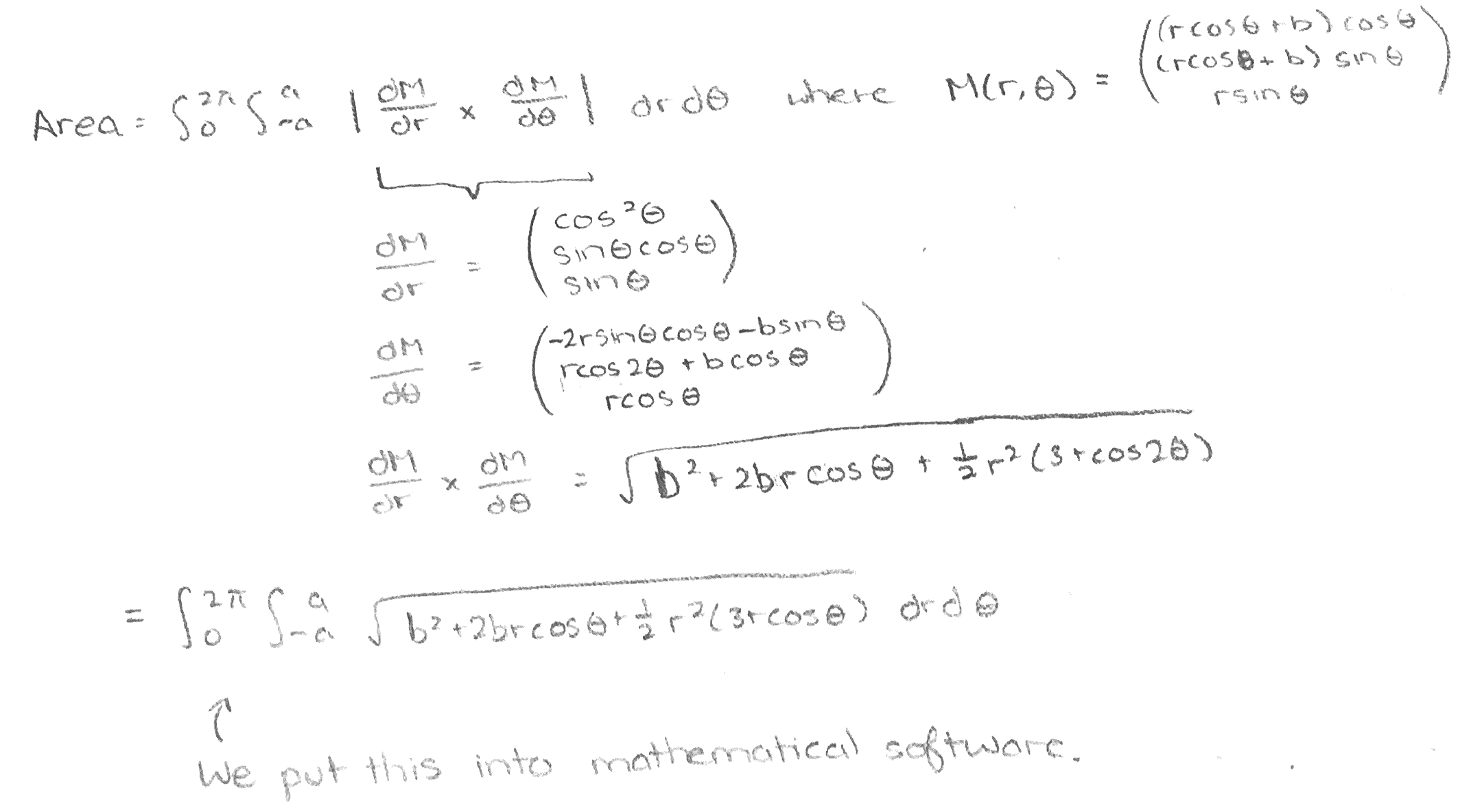(For a more thorough explanation of the calculus behind parametrizing a mobius strip and calculating surface area, see https://www.mathstat.dal.ca/~antoniov/bagelrings.html).

The surface area of a straight cut through the center of bagel is more straightforward. If you’ve ever split open a bagel the normal way, you’ll likely have noticed that the exposed bagel is simply a circle with a hole in the middle. Therefore, we can find this surface area just by taking the area of the outer circle (π(a+b)^2) and subtracting the area of the smaller circle (π(a-b)^2).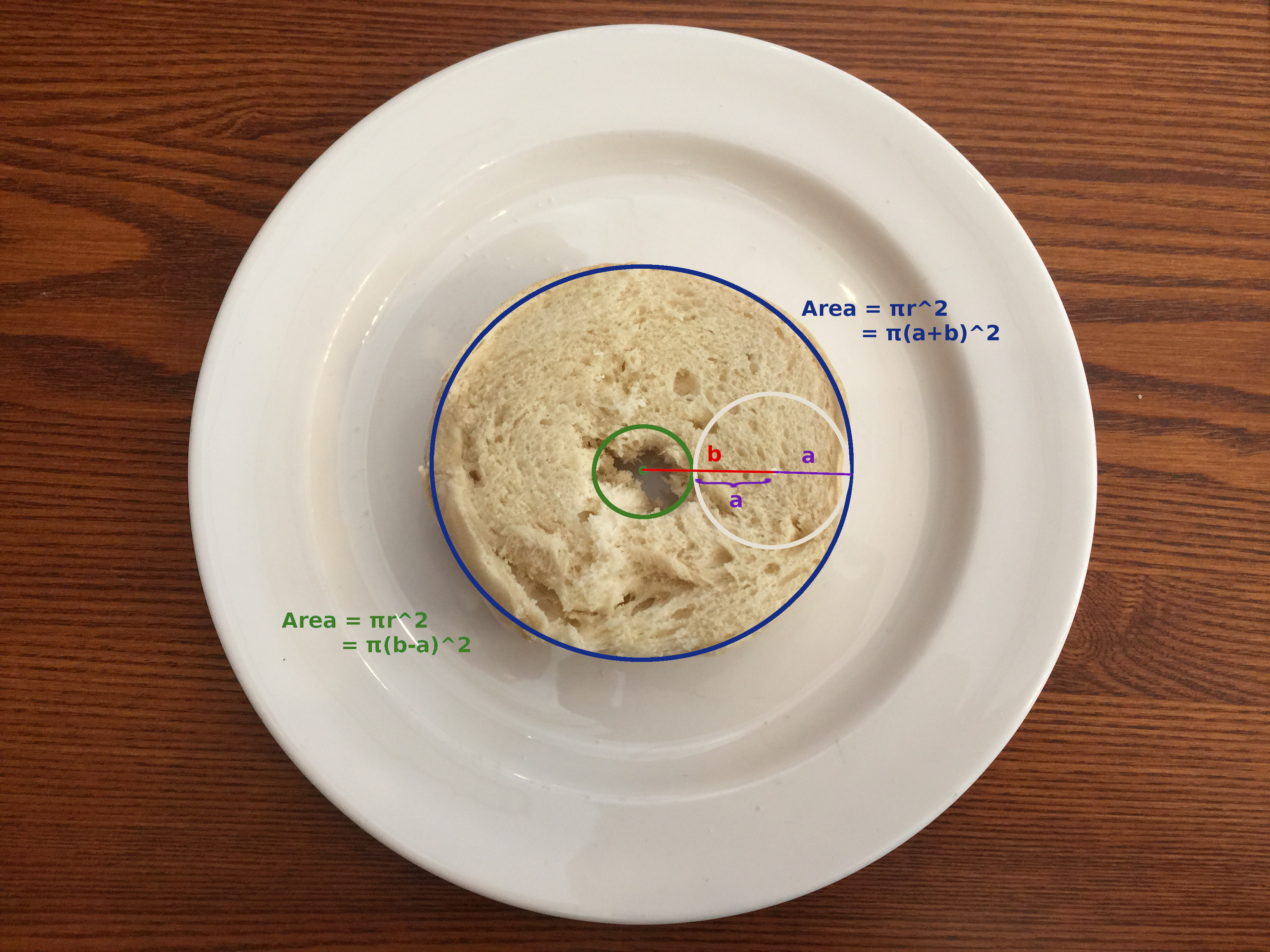Comparing the two areas using mathematical software, we find that the ratio of the surface area of the mobius cut to the surface area of the flat cut only changes when we change the ratio between a and b. Additionally, that ratio of surface areas is always greater than 1, so we can guarantee that the mobius strip will always give more surface area! However, the question of how much additional “bagelscape” we have for our cream cheese is dependent on the characteristics of the bagel shape. The greater a is in comparison to b, the greater the ratio of surface areas. That is, the fatter the bagel and the smaller the hole, the more benefit we get out of cutting our bagel using the mobius strip!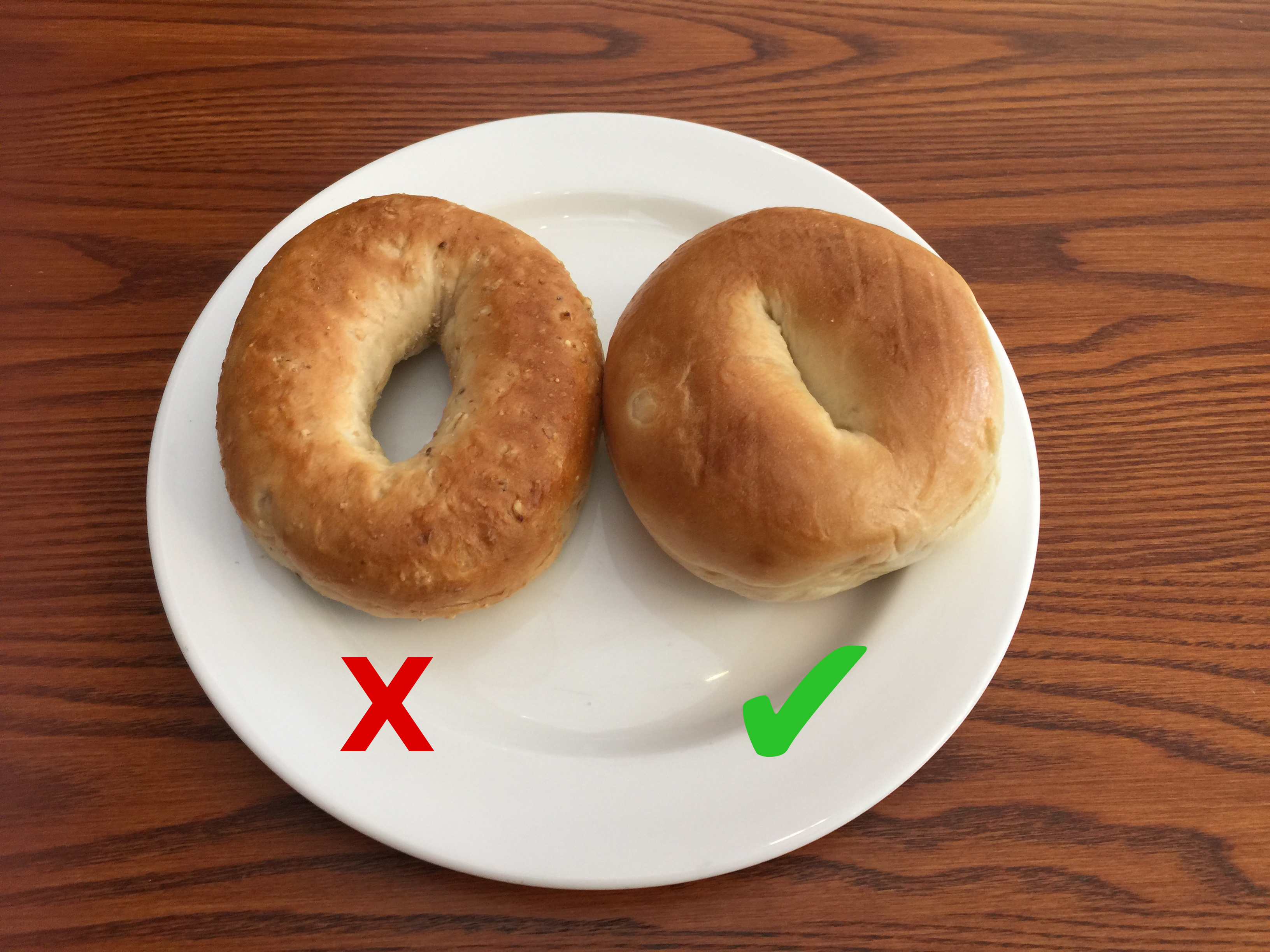In theory, the shape of the bagel on the left will give less significant gains in surface area from using a mobius cut while the shape of the one on the right will give more gains out of using a mobius cut.

However, we run into a significant physical reality check when we realize that for extremely thick bagels, the cut will not work because we cannot fit the bagel through its own center hole. Therefore, we restrict

2a < 2(b-a)

2a < 2b – 2a

4a < 2b

a < b/2

Therefore, referring to our previous diagram of the torus, the Mobius cut will only work for a<b/2, in which case the surface area gain is sadly only about 5% from our mathematical software analysis.

Resources and Acknowledgements:

Hart, George W. “Mathematically Correct Breakfast: How to slice a bagel into two linked halves.” https://www.georgehart.com/bagel/bagel.html

Vargas, Antonio R. “On calculating a ‘Mathematically Correct Breakfast’.” (2014). Dalhousie University. https://www.mathstat.dal.ca/~antoniov/bagelrings.html

Weisstein, Eric W. “Torus.” From MathWorld–A Wolfram Web Resource. http://mathworld.wolfram.com/Torus.html